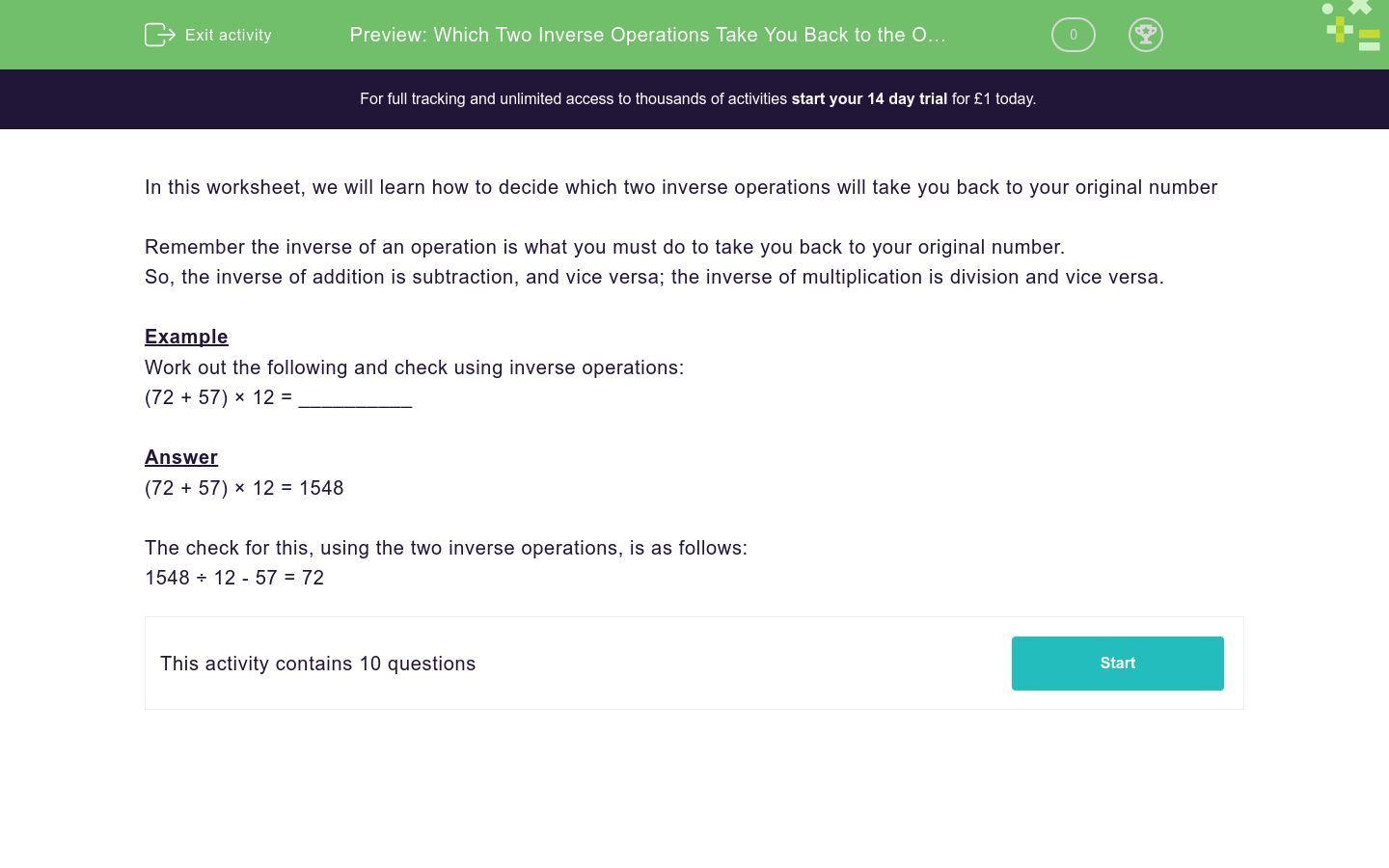# Which Two Inverse Operations Take You Back to the Original Number?

In this worksheet, students work out an answer and then decide which two inverse operations will take them back to the original number.Key stage:  KS 3

Curriculum topic:   Number

Curriculum subtopic:   Use Relationships Between Operations

Difficulty level:### QUESTION 1 of 10

In this worksheet, we will learn how to decide which two inverse operations will take you back to your original number

Remember the inverse of an operation is what you must do to take you back to your original number.

So, the inverse of addition is subtraction, and vice versa; the inverse of multiplication is division and vice versa.

Example

Work out the following and check using inverse operations:

(72 + 57) × 12 = __________

(72 + 57) × 12 = 1548

The check for this, using the two inverse operations, is as follows:

1548 ÷ 12 - 57 = 72

Work out the following and check using inverse operations:

81 × 6 + 87 = ___________

Work out the following and check using inverse operations:

(40 + 18) × 7 = ___________

Work out the following and check using inverse operations:

82 × 7 + 40 = ___________

Work out the following and check using inverse operations:

64 × (3 + 18) = ___________

Work out the following and check using inverse operations:

(99 + 91) × 12 = ___________

Work out the following and check using inverse operations:

(89 - 65) ÷ 6 = ___________

Work out the following and check using inverse operations:

(95 + 82) × 8 = ___________

Work out the following and check using inverse operations:

168 ÷ 12 + 54 = ___________

Work out the following and check using inverse operations:

(42 + 70) ÷ 7 = ___________

Work out the following and check using inverse operations:

(45 + 93) × 6 = ___________

• Question 1

Work out the following and check using inverse operations:

81 × 6 + 87 = ___________

573
EDDIE SAYS
Inverse check is (Answer - 87) ÷ 6 = 81
• Question 2

Work out the following and check using inverse operations:

(40 + 18) × 7 = ___________

406
EDDIE SAYS
Inverse check is (Answer ÷ 7) -18 = 40
• Question 3

Work out the following and check using inverse operations:

82 × 7 + 40 = ___________

614
EDDIE SAYS
Inverse check is (Answer - 40) ÷ 7 = 82
• Question 4

Work out the following and check using inverse operations:

64 × (3 + 18) = ___________

1344
EDDIE SAYS
Inverse check is (Answer ÷ 64) -3 = 18
• Question 5

Work out the following and check using inverse operations:

(99 + 91) × 12 = ___________

2280
EDDIE SAYS
Inverse check is (Answer ÷ 23) -91 = 99
• Question 6

Work out the following and check using inverse operations:

(89 - 65) ÷ 6 = ___________

4
EDDIE SAYS
Inverse check is (Answer x 6) + 65 = 89
• Question 7

Work out the following and check using inverse operations:

(95 + 82) × 8 = ___________

1416
EDDIE SAYS
Inverse check is (Answer ÷ 8) -82 = 95
• Question 8

Work out the following and check using inverse operations:

168 ÷ 12 + 54 = ___________

68
EDDIE SAYS
Inverse check is (Answer - 54) x 12 = 168
• Question 9

Work out the following and check using inverse operations:

(42 + 70) ÷ 7 = ___________

16
EDDIE SAYS
Inverse check is (Answer x 7) - 70 = 42
• Question 10

Work out the following and check using inverse operations:

(45 + 93) × 6 = ___________

828
EDDIE SAYS
Inverse check is (Answer ÷ 6) - 93 = 45
---- OR ----

Sign up for a £1 trial so you can track and measure your child's progress on this activity.

### What is EdPlace?

We're your National Curriculum aligned online education content provider helping each child succeed in English, maths and science from year 1 to GCSE. With an EdPlace account you’ll be able to track and measure progress, helping each child achieve their best. We build confidence and attainment by personalising each child’s learning at a level that suits them.

Get started